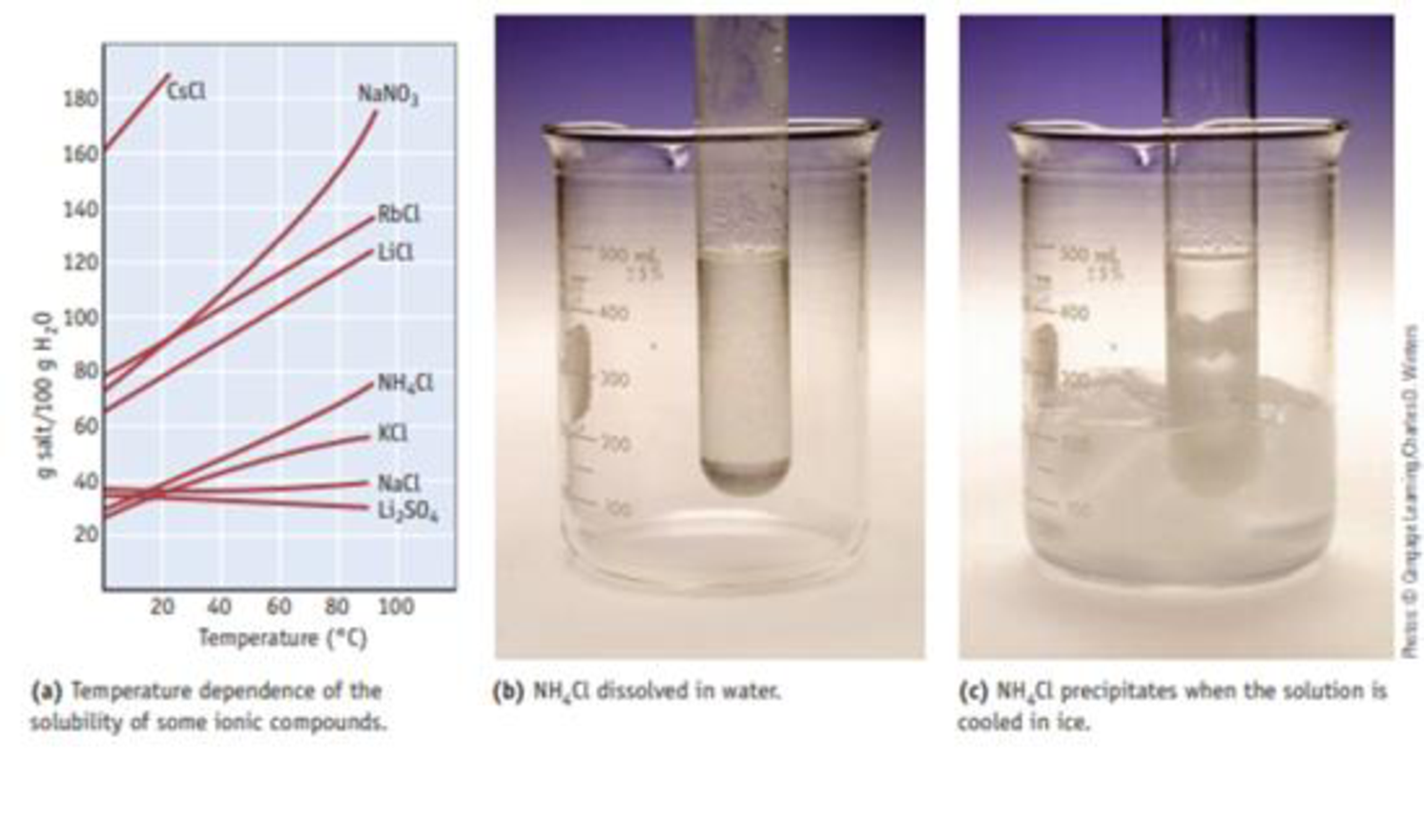Chapter 13, Problem 17PS

Chapter
Section
Textbook Problem

You make a saturated solution of NaCl at 25 °C. No solid is present in the beaker holding the solution. What can be done to increase the amount of dissolved NaCl in this solution? (See Figure 13.11.) (a) Add more solid NaCl. (b) Raise the temperature of the solution. (c) Raise the temperature of the solution, and add some NaCl. (d) Lower the temperature of the solution, and add some NaCl.Figure 13.11 The temperature dependence of the solubility of some ionic compounds in wafer. The solubility of most ionic compounds increases with increasing temperature This is illustrated using NH4CI (ports b and c).

Interpretation Introduction

Interpretation: The method to increase the amount of dissolved salt in the solution has to be identified.

Concept introduction:

Enthalpy of solution: In the process, the change in enthalpy while dissolving the solute in the solvent.

Explanation

Reason for correct answer:

Option c):

Raising the temperature increases the solubility of solid by further addition of NaCl.

Hence, the statement is true.

Reason for wrong answers:

Option a):

Adding more solid, the saturation level increases but the salt gets dissolved at slow rate

Still sussing out bartleby?

Check out a sample textbook solution.

See a sample solution

The Solution to Your Study Problems

Bartleby provides explanations to thousands of textbook problems written by our experts, many with advanced degrees!

Get Started

Find more solutions based on key concepts